## Mandala Building Blocks - 2

This activity has the following desired goals:

• Learning about the different pre-defined mandala building blocks (A, M).

### Building Block 5 - Semi Circular Petal

Type in the following code and run it:

``````def semiCircularPetal(radius: Double, theta: Double, thetaExtent: Double) = Picture.fromVertexShape { s =>
val tDelta = thetaExtent / 2
import s._
beginShape()

curveVertexRt(radius + (0.7010526 * thetaExtent), theta - tDelta / 2)

curveVertexRt(radius + (0.93333 * thetaExtent), theta)

curveVertexRt(radius + (0.7010526 * thetaExtent), theta + tDelta / 2)

endShape()
}

cleari()
showAxes()
showGrid()
val pic = semiCircularPetal(150, 0, 80)
draw(pic)
``````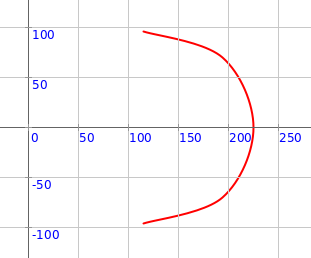Q5a. The code above makes one semi circular petal. What is its radius? What is its direction (theta)? What is it’s spread (thetaExtent)?

#### Exploration

Play with the inputs to the `semiCircularPetal` function above to make different kinds of petals.

#### Exercise

Make the following circular pattern: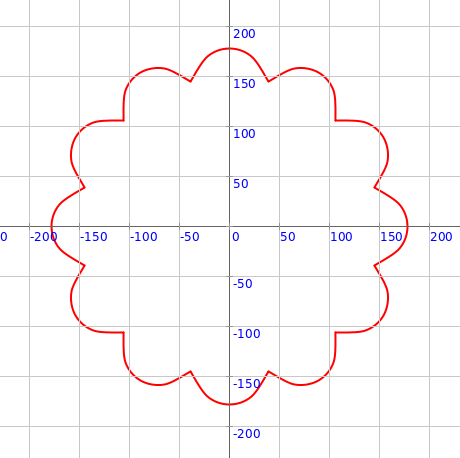### Building Block 6 - Inscribed Triangle

Type in the following code and run it:

``````def inscribedTriangle(vertexR: Double, vertexTheta: Double) = Picture.fromVertexShape { s =>
import s._
beginShape()
vertexRt(vertexR, vertexTheta)
vertexRt(vertexR, vertexTheta + 120)
vertexRt(vertexR, vertexTheta + 240)
vertexRt(vertexR, vertexTheta)
endShape()
}

cleari()
showAxes()
showGrid()
val pic = inscribedTriangle(150, 0)
draw(pic)
``````Q6a. The code above makes one inscribed triangle. What is its circumscribing radius? What is its direction (theta)?

#### Exploration

Play with the inputs to the `inscribedTriangle` function above to make different kinds of triangles.

#### Exercise

Make the following circular pattern: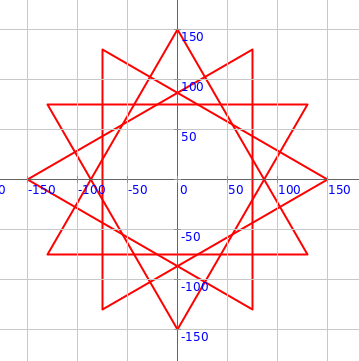### Building Block 7 - Inscribed Square

Type in the following code and run it:

``````def inscribedSquare(vertexR: Double, vertexTheta: Double) = Picture.fromVertexShape { s =>
import s._
beginShape()
vertexRt(vertexR, vertexTheta)
vertexRt(vertexR, vertexTheta + 90)
vertexRt(vertexR, vertexTheta + 180)
vertexRt(vertexR, vertexTheta + 270)
vertexRt(vertexR, vertexTheta)
endShape()
}

cleari()
showAxes()
showGrid()
val pic = inscribedSquare(150, 0)
draw(pic)
``````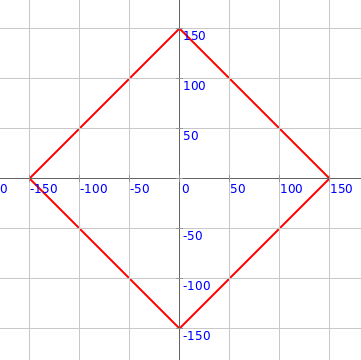Q7a. The code above makes one inscribed square. What is its circumscribing radius? What is its direction (theta)?

#### Exploration

Play with the inputs to the `inscribedSquare` function above to make different kinds of squares.

#### Exercise

Make the following circular pattern: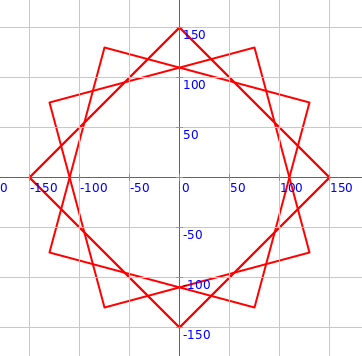### Building Block 8 - Altar

Type in the following code and run it:

``````def altar(r: Double, gateRFraction: Double, gateTheta: Double) = Picture {
hop(r)
left(90)
val glen = r * gateRFraction
hop(glen)
repeat(4) {
forward(r - glen)
left()
forward(r - glen)
right()
forward(r / 10)
right()
forward(r / 4)
left()
forward(r / 10)
left()
forward(r / 4)
forward(glen * 2)
forward(r / 4)
left()
forward(r / 10)
left()
forward(r / 4)
right()
forward(r / 10)
right(90)
}
}

cleari()
showAxes()
showGrid()
val pic = altar(200, 0.1, 0)
draw(pic)
``````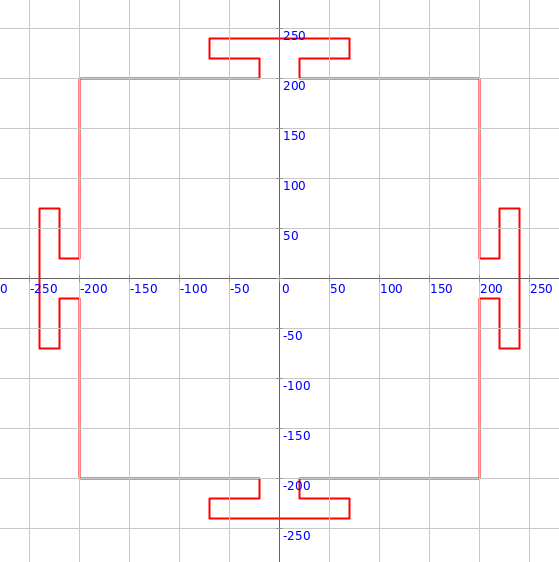Q8a. The code above makes an altar. What is its inscribing radius? What is its direction (theta)?

#### Exploration

Play with the inputs to the `altar` function above to make different kinds of altars.

#### Exercise

Make the following circular pattern: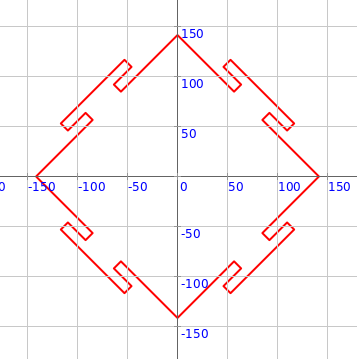More building blocks coming soon…